### CAT 2018 Question Paper (Slot 1) Question 59

Instructions

1600 satellites were sent up by a country for several purposes. The purposes are classified as broadcasting (B), communication (C), surveillance (S), and others (O). A satellite can serve multiple purposes; however a satellite serving either B, or C, or S does not serve O. The following facts are known about the satellites:

1. The numbers of satellites serving B, C, and S (though may be not exclusively) are in the ratio 2:1:1.
2. The number of satellites serving all three of B, C, and S is 100.
3. The number of satellites exclusively serving C is the same as the number of satellites exclusively serving S. This number is 30% of the number of satellites exclusively serving B.
4. The number of satellites serving O is the same as the number of satellites serving both C and S but not B.

Question 59

# What best can be said about the number of satellites serving C?

Solution

It is given that a satellite serving either B, or C, or S does not serve O. So we can say that it's basically 3 satellites broadcasting (B), communication (C), surveillance (S) which can have intersections. Those satellites which are not part of any category are placed in others. We can draw the Venn diagram as follows.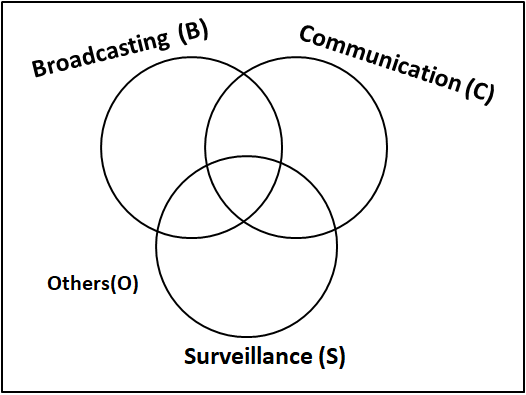1. The numbers of satellites serving B, C, and S (though may be not exclusively) are in the ratio 2:1:1.
2. The number of satellites serving all three of B, C, and S is 100.
3. The number of satellites exclusively serving C is the same as the number of satellites exclusively serving S. This number is 30% of the number of satellites exclusively serving B.
4. The number of satellites serving O is the same as the number of satellites serving both C and S but not B.

Let '10x' be the number of satellites exclusively serving B. Then, the number of satellites exclusively serving C and S = 0.30*10x = 3x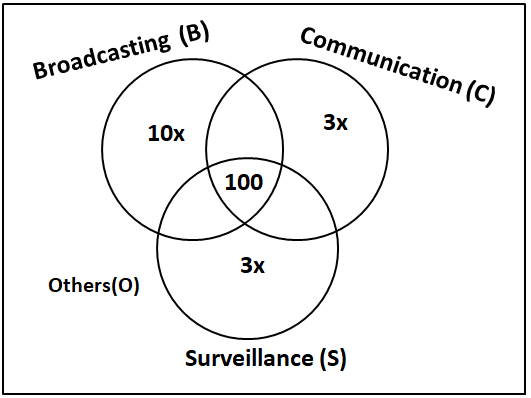Let 'y' be the number of satellites serving others(O).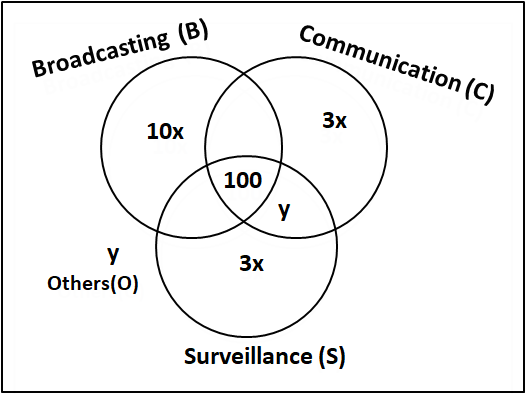Let 'z' be the number of satellites serving B, C but not S. Since the numbers of satellites serving B, C, and S (though may be not exclusively) are in the ratio 2:1:1. Therefore, we can can say that number of satellites serving B, S but not C = z.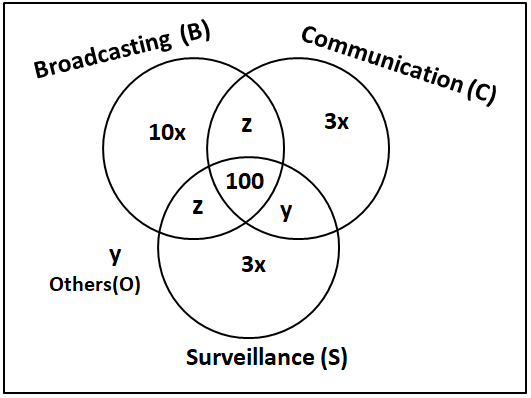It is given that

$$\Rightarrow$$ 10x+2z+2y+6x+100 = 1600

$$\Rightarrow$$ 8x+z+y = 750 ... (1)

The numbers of satellites serving B, C, and S (though maybe not exclusively) are in the ratio 2:1:1.

$$\Rightarrow$$ $$\dfrac{10x+2z+100}{z+100+3x+y} = \dfrac{2}{1}$$

$$\Rightarrow$$ $$10x+2z+100=2(z+100+3x+y)$$

$$\Rightarrow$$ $$4x=100+2y$$

$$\Rightarrow$$ $$2x=50+y$$

$$\Rightarrow$$ $$y=2x-50$$   ... (2)

We can substitute this in equation (1)

$$\Rightarrow$$ 8x+z+2x - 50 = 750

$$\Rightarrow$$ z = 800 - 10x    ... (3)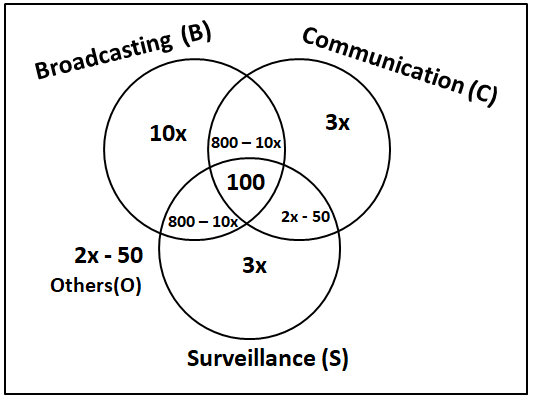Let us define boundary condition for x,

$$\Rightarrow$$ 2x - 50 $$\geq$$ 0

$$\Rightarrow$$ x $$\geq$$ 25

Also,  800 - 10x $$\geq$$ 0

$$\Rightarrow$$ x $$\leq$$ 80

Therefore, we can say that x $$\epsilon$$ [25, 80].

The number of satellites serving C = 800 - 10x + 100 + 3x + 2x - 50 = 850 - 5x

At x = 25, The number of satellites serving C = 850 - 5x = 850 - 5*25 = 725

At x = 80, The number of satellites serving C = 850 - 5x = 850 - 5*80 = 450

Hence, we can say that the number of satellites serving C must be between 450 and 725. Hence, option C is the correct answer.